# Antiderivative facts for kids

Kids Encyclopedia Facts

Antidifferentiation (also called indefinite integration) is a part of mathematics. It is the opposite of differentiation. Antidifferentiation is integration with no limits (which is why it is called indefinite). The answer to an antiderivative is an equation.

It is written as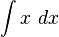$\int x\ dx$

• With the integral sign that has no limits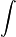$\int$
• The equation you are integrating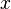$x$
• And the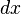$dx$ which means "with respect to$x$", which does not mean anything with simple integration.

## Simple integration

To integrate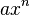$ax^n$

• Add 1 to the power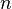$n$, so$ax^n$ is now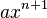$ax^{n+1}$
• Divide all this by the new power, so it is now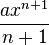$\frac{ax^{n+1}}{n+1}$
• Add constant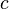$c$, so it is now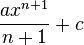$\frac{ax^{n+1}}{n+1} + c$

This can be shown as: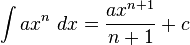$\int ax^n\ dx = \frac{ax^{n+1}}{n+1} + c$

When there are many$x$ terms, integrate each part on its own: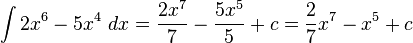$\int 2x^6 - 5x^4\ dx = \frac{2x^7}{7} - \frac{5x^5}{5} + c = \frac{2}{7}x^7 - x^5 + c$

(This only works if the parts are being added or taken away.)

### Examples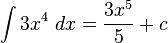$\int 3x^4\ dx = \frac{3x^5}{5} + c$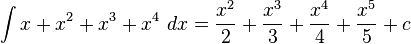$\int x + x^2 + x^3 + x^4\ dx = \frac{x^2}{2} + \frac{x^3}{3} + \frac{x^4}{4} + \frac{x^5}{5} + c$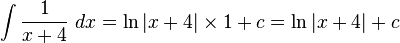$\int \frac{1}{x + 4}\ dx = \ln |x + 4| \times 1 + c = \ln |x + 4| + c$

Changing fractions and roots into powers makes it easier: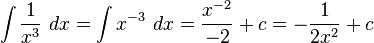$\int \frac{1}{x^3}\ dx = \int x^{-3}\ dx = \frac{x^{-2}}{-2} + c = -\frac{1}{2x^2} + c$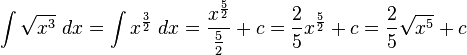$\int \sqrt{x^3}\ dx = \int x^{\frac{3}{2}}\ dx = \frac{x^{\frac{5}{2}}}{\frac{5}{2}} + c = \frac{2}{5}x^{\frac{5}{2}} + c = \frac{2}{5}\sqrt{x^5} + c$

## Integrating a bracket ("chain rule")

If you want to integrate a bracket like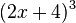$(2x+4)^3$, we need to do it a different way. It is called the chain rule. It is like simple integration. It only works if the$x$ in the bracket has a power of 1 (it is linear) like$x$ or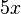$5x$ (not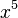$x^5$ or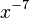$x^{-7}$).

To do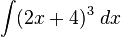$\int (2x+4)^3\ dx$

• Add 1 to the power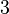$3$, so that it is now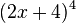$(2x+4)^4$
• Divide all this by the new power to get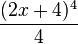$\frac{(2x+4)^4}{4}$
• Divide all this by the derivative of the bracket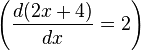$\left (\frac{d(2x+4)}{dx} = 2 \right )$ to get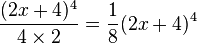$\frac{(2x+4)^4}{4 \times 2} = \frac{1}{8}(2x+4)^4$
• Add constant$c$ to give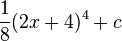$\frac{1}{8}(2x+4)^4 + c$

### Examples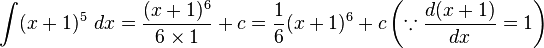$\int (x+1)^5\ dx = \frac{(x+1)^6}{6 \times 1} + c = \frac{1}{6}(x+1)^6 + c \left ( \because \frac{d(x+1)}{dx} = 1 \right )$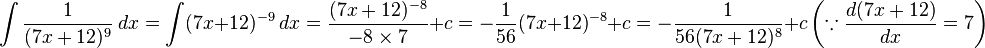$\int \frac{1}{(7x+12)^9}\ dx = \int (7x+12)^{-9}\ dx = \frac{(7x+12)^{-8}}{-8 \times 7} + c = -\frac{1}{56}(7x+12)^{-8} + c = -\frac{1}{56(7x+12)^8} + c \left ( \because \frac{d(7x+12)}{dx} = 7 \right )$

## Images for kidsAntiderivative Facts for Kids. Kiddle Encyclopedia.